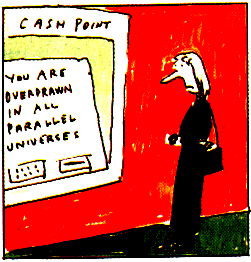MR1277678 (95d:83011)
Alcubierre, Miguel(4-WALC-PA)
The warp drive: hyper-fast travel within general relativity. (English. English summary)
Classical Quantum Gravity 11 (1994), no. 5, L73--L77.
83C15 (83C10)
In Minkowski space, if two objects $A$ and $B$ are separated by a proper spatial distance $D$, then it is impossible for anything travelling no faster than light to get from $A$ to $B$ in a time less than $D/c$, where the time is as measured in the frame of reference in which the two objects are at rest. The author shows that it is possible to construct a new metric which has the following features: (1) the spatial geometry of the surfaces of constant $t$ is unchanged, i.e. they remain flat, (2) there is a timelike geodesic connecting $A$ to $B$, (3) proper time along the geodesic coincides with coordinate time, so that the time elapsed as measured by a stationary observer coincides with that for an observer who travels along the geodesic, and (4) the space-time is flat outside a neighbourhood of the timelike geodesic from $A$ to $B$. Unfortunately, the space-time also violates the weak energy condition, and so its physicality is problematic.

Reviewed by Robert J. Low

Sounds good! However,...
MR1672464 (2000a:83047)
Low, Robert J.(4-COVE-MM)
Speed limits in general relativity. (English. English summary)
Classical Quantum Gravity 16 (1999), no. 2, 543--549.
83C40 (83C99)
Einstein's field equation is considered, restricting to matter models whose energy-momentum tensor depends only on the spacetime metric and on a vector field $u$. Such a matter model is called physically reasonable if the energy-momentum tensor satisfies the dominant energy condition and the vector field $u$ satisfies a quasi-linear symmetric hyperbolic evolution equation, otherwise it is called exotic. The main goal of the paper is to show that, within the indicated class of matter models, it is impossible to construct a warp drive as considered by M. Alcubierre \ref[Classical Quantum Gravity 11 (1994), no. 5, {\rm L}73--{\rm L}77; MR1277678 (95d:83011)] in the absence of exotic matter.

Reviewed by Volker Perlick

What can we do now...?

Is FTL really a contradiction to GRT? At least, by using group properties of the LT and a bit linear algebra, one can construct a SRT without *assuming* the existence of a constant velocity... Of course, this can then be derived, but no one claims that this constant velocity is really the light velocity $c$...!? Any - deliberate - comment (by e-mail) is highly appreciated!Current Version: September 5, 2005